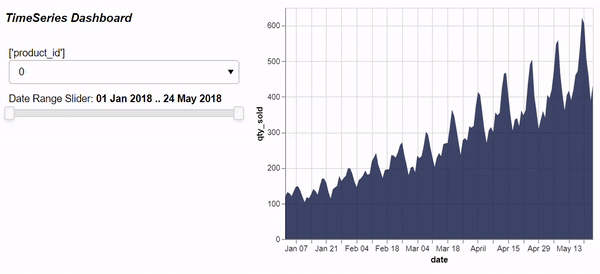# Can't render Altair Chart based on DateSelectSlider

Hi! My struggle with Altair and Panel widget continue, I’m trying to create an Altair chart and control It with different widgets, at first stage using a Date Select Slider, based on this tutorial (https://towardsdatascience.com/how-to-build-a-time-series-dashboard-in-python-with-panel-altair-and-a-jupyter-notebook-c0ed40f02289) code I tried to build this function as first step:

`
#The Dashboard Function

``````import pandas as pd
import panel as pn
import numpy as np
import altair as alt
from vega_datasets import data
from dateutil.parser import parse
from altair import datum
def ts_dashboard(dtf, resolution, date_col, col_to_analyse):
title = '### *_TimeSeries Dashboard_*'
for res in resolution:
tickers = dtf[res].unique()
ticker = pn.widgets.Select(name=str(resolution), options=list(tickers))
date_range_slider = pn.widgets.DateRangeSlider(
name='Date Range Slider',
start=parse(dtf[date_col]), end=parse(dtf[date_col][len(dtf) - 1]),
value=(parse(dtf[date_col]), parse(dtf[date_col][len(dtf) - 1]))
)
@pn.depends(ticker.param.value, date_range_slider.param.value)
def get_plot(ticker_val, data_range):
data = dtf
data[date_col] = pd.to_datetime(data[date_col])
start_date = data_range
end_date = data_range
mask = (data[date_col] > start_date) & (data[date_col] <= end_date)
# pylint:disable=(undefined-loop-variable)
chart = alt.Chart(data).mark_area(color="#0c1944", opacity=0.8)\
.encode(x=date_col, y=col_to_analyse,
tooltip=alt.Tooltip([date_col, col_to_analyse]))\
.transform_filter((datum[res] == ticker_val))
return chart
return get_plot, title, ticker, date_range_slider

#Test DataFrame

source = data.stocks()
source = pd.DataFrame(source)
#Panel Object and Deployement

plot, title, ticker, date_range_slider =ts_dashboard(source,['symbol'],'date','price')
dashboard=pn.Row(pn.Column(title, ticker,date_range_slider),
plot).show()`
``````

It works just fine in Jupyter Notebook, but unfortunately , using Pycharm the Chart does not interact with the date slider, as shown below :I would like to integrate this dashboard as part pf my panel App, and I’m using Pycharm to do so, but I don’t really know what I missed up, I cheked the pachages version, and they are the same in JupyterNootebook and Pycharm, I also used alt.renderers.enable(‘altair_viewer’), as suggested in Altair Doc as well but nothing seem to work, I’m really confused about this, could you guide me please